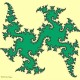# Low Dimensional Topology

## March 13, 2008

### Group theory, as told by a Heegaard splitter, Part 3

Filed under: 3-manifolds,Heegaard splittings,Mapping class groups — Jesse Johnson @ 11:54 am

Ok, one more post about group theory and then I’ll drop it. Ben Webster asked a question on my last mapping class group post about whether you could think about mapping class groups of Heegaard splittings in terms of actions on the fundamental group of the 3-manifold. Well it happens that almost any question about Heegaard splittings can be studied in terms of fundamental groups without even knowing what a Heegaard splitting is, though you need to look at more than just the fundamental group of the 3-manifold.

Stallings  introduced the idea of a splitting homomorphism as follows: Consider two homomorphisms from a genus g surface fundamental group onto two rank g free groups. Consider the product of the kernels of these two homomorphisms in the surface group. The product is a normal subgroup so we can take the quotient G of the surface group by this product. There are induced homomorphisms from the two free groups into G, making a commutative diamond.

Jaco showed  that for any two such homomorphisms, there is a way of identifying a genus g surface with the boundaries of two handlebodies, forming a 3-manifold and a Heegaard splitting for that 3-manifold, such that the inclusion maps between the fundamental groups are the two homomorphisms we started with. It follows that G is the fundamental group of the 3-manifold. Given two splitting homomorphisms (i.e. commutative diamonds of this form), we can consider a collection of four homomorphisms between corresponding elements forming a commutative cube. Such a cube corresponds to a homeomorphism between the two 3-manifolds that takes one Heegaard splitting to the other. Thus two splitting homomorphisms form a commutative cube if and only if they correspond to homeomorphic Heegaard splittings of homeomorphic 3-manifolds.

One might want to determine whether two Heegaard splittings are isotopic rather than just homeomorphic. In this case, one can consider two splitting diagrams that share the same bottom group (i.e. the fundamental group of the 3-manifold). This is sort of a commutative pair of glasses. If there are homomorphisms between the three remaining pairs of corresponding groups that make a commutative diagram (a squished cube?) then the corresponding Heegaard splittings are (in most cases) isotopic. (By “most cases” I mean for the large class of 3-manifolds for which an isomorphism between fundamental groups corresponds to a unique homeomorphism.)

So this gives one a completely algebraic way to study homeomorphism classes and isotopy classes of Heegaard splittings. The mapping class group of a Heegaard splitting is the set of commutative cubes between a fixed splitting homomorphism and itself. (Multiplication and inverses are easy to work out.) Jaco describes in  a construction on the commutative diamond that corresponds to stabilizing the Heegaard splitting.

Now that I’ve described all this, I should point out that it’s probably not a very useful approach to Heegaard splittings. As far as I know there are only four or five papers in existence on splitting diagrams, and though things have been proved about them, nothing has been proved using them. But who knows, maybe someone who knows more about group theory than the average Heegaard splitter could find something new.

 Stallings, John. How not to prove the Poincare conjecture. Topology Seminar, Wisconsin, 1965, Ann. of Math. Studies, No. 60 (Princeton 1966).

 Jaco, William. Heegaard splittings and splitting homomorphisms. Trans. Amer. Math. Soc. 144 1969 365–379.

 Jaco, William. Stable equivalence of splitting homomorphisms. 1970 Topology of Manifolds (Proc. Inst., Univ. of Georgia, Athens, Ga., 1969) pp. 153–156 Markham, Chicago, Ill.

## 1 Comment »

1.Indeed, Makanin ignored Stallings’ advice and recently posted a paper purporting to prove the Poincare conjecture using the splitting homomorphisms:
http://front.math.ucdavis.edu/0711.3706
The paper is difficult to read, partly because he seems to have used MS Word to produce it.
Makanin is an expert on geometric group theory and equations over the free group
(he developed Makanin-Razborov diagrams which are a useful technical tool for studying
homomorphisms to free groups).

Comment by Ian Agol — March 14, 2008 @ 7:35 pm

Blog at WordPress.com.